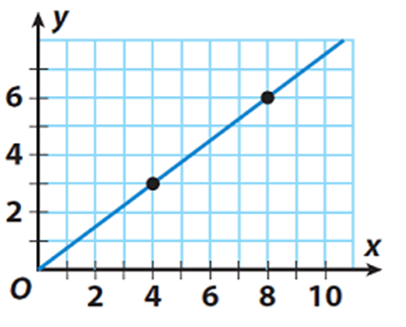SherpaMaths

>

GCSE

Rates of Change

Question

# How do you find the rate of change?

1 year ago

·

126 Replies

·

4402 views

V

Vickie Shanahan

C
Charlie

To find the rate of change, you must divide the change in y by the change in x (if using a graph, where y is the vertical axis and x is the horizontal axis).

L
Leon Cragg

the change in y-values by the change in x-values.

S
Sonya Pervez

The rate of change (ROC) is how much the value of something changes over a period of time and it is given in percentage terms.

To find this you first calculate the change in "Y" values (or the change in an outcome variable) divided by the change in "X" values (or the change in input variables).

So if my tutoring time increases from 3 hours in week 1 to 16 hours in week 2 and my income increases from £45 per week to £240 per week, Y values are my income and X values are the work hours I put in. r

To calculate the ROC I would do the following.

(240 - 45) / (16 - 3) = 195 / 13 = 15% rate of change in my earnings between week 1 and week 2.

DR M Verified Sherpa Tutor ✓

An experienced tutor for science subjects and competitive exams

If you take the change in Y values and divide that with the change in X-values the result will be a rate of change,

I'm available for 1:1 private online tuition!

Vashisht K Verified Sherpa Tutor ✓

4 years teaching GCSE. Engineering Student at King's College London

2 reviews

In a graph of a value vs time, it would be the gradient. Otherwise simply divide the difference in any particular value by the time taken.

I'm available for 1:1 private online tuition!

O
Omar Choudhry

Rate of change = (Change in quantity 1) / (Change in quantity 2)

T
Timothy M Lewis

Assuming rate of charge is the rate of flow of charge, this is known as current (I). This can be found by dividing charge (Q) by time (t) - I = Q/t

D
Darcy Peake

The rate of change refers to how much something for example distance is changing in a specific unit of time. In order to work out the rate of change, you must divide the thing that is changing with how much it has changed in such a unit of time.

Krish S Verified Sherpa Tutor ✓

Dedicated, Experienced Maths & Science Tutor

Hi Mrs Shanahan,

Rate of change is also known as the gradient. You can either use calculus to find the rate of change - this would be differentiation - and there are a range of methods you can use. These should be listed in your specification either in IGCSE, further maths GCSE, or A-Level.

A non-calculus method, more commonly known to those who are not studying at A-Level, is to simply estimate the rate of change. You can do this by taking two points on a linear graph (x1, y1) and (x2, y2) and finding the change in y / change in x. I.e. (y2-y1)/(x2-x1). For a non-linear graph, you can do the same by drawing a tangent at the point you want to find the rate of change and applying the same logic as above.

Hope this helps.

I'm available for 1:1 private online tuition!

Fyruz N Verified Sherpa Tutor ✓

My teaching is based on the individual learning system of the student.

rate of change can be found by using the equation - Difference in Y/ Difference in X

I'm available for 1:1 private online tuition!

N
NEHA GUPTA

By the help of differentiation

H
Hank

If we get the explicit equation, we could just differentiate that wrt the variable. If we don’t, we can estimate it by calculating the change on the y-axis and dividing it by the change on the x-axis.

S
Stavros Savva

Divide the change of one variable with the change of the other variable

L
Lewis Lovell

Hello Mrs Vickie Shanahan. There isn't much context to your question here, so I'll keep my response as general as possible:

The rate of change describes a relationship between a dependent and an independent variable. For example, the rate of change of velocity with time or the rate of change of temperature with distance.

In a simple mathematical case, we'd write this as the change in the dependent variable (temperature, dT) over a fixed interval of the independent variable (distance, dx)

Which is mathematically written as dT/dx, or as shown in the graph, dy/dx.It is then clear that for the linear relationship in the above graph, the rate of change is dy/dx = (y1-y2)/(x2-x1) = (6-3)/(8-4).

U
Unai Llona Carbajo

Derivatives are the best tool, but not for everyone... Considering two variables (distance and time, for example), you can also take the first and last value of both in a certain process, subtract the ones from the same variable (e.g. last and first distances of the bus from your position) and divide the subtractions of different variables. By doing this with position/distance and time, the rate of change you get is the speed of the object you're analyzing!

Think you can help?## Need a GCSE Maths tutor?

Get started with a free online introductions with an experienced and qualified online tutor on Sherpa.

Find a GCSE Maths Tutor